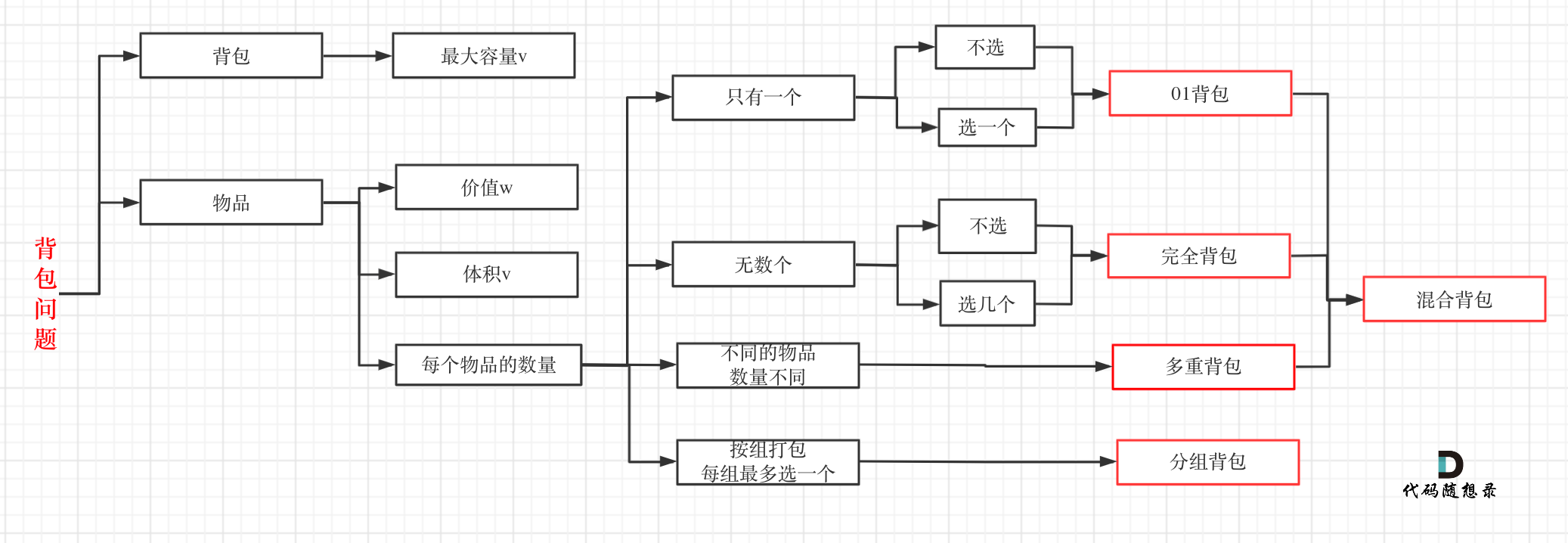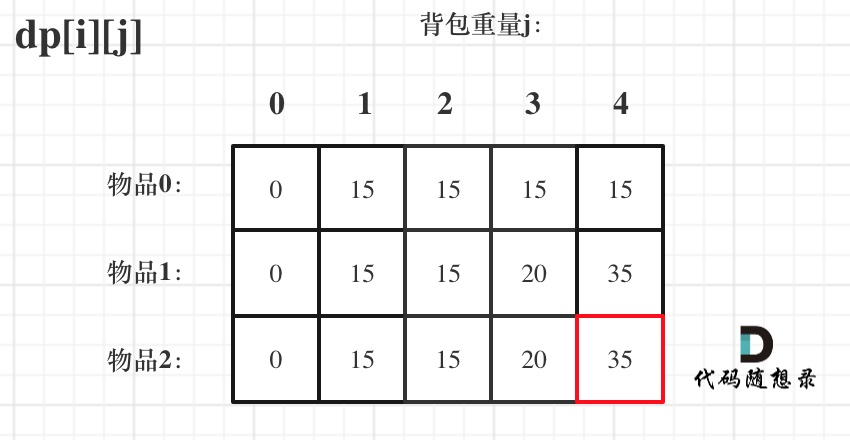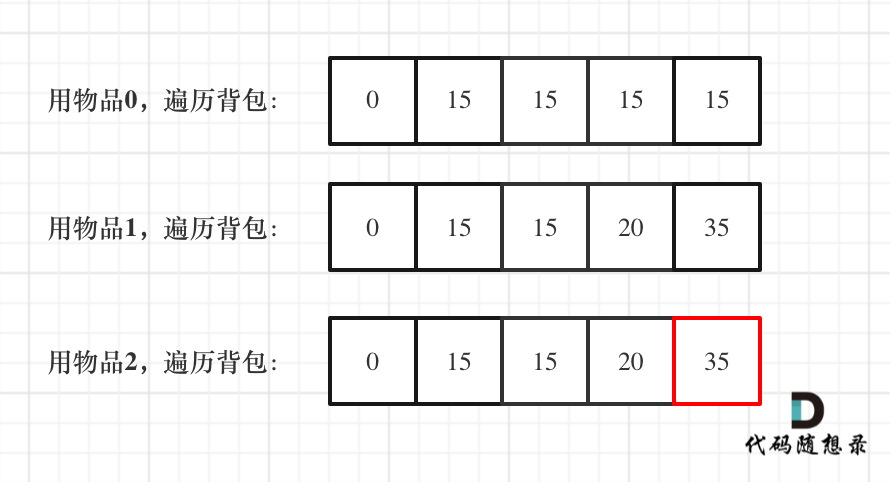# 本周小结！（动态规划系列三）

# 周一1. 确定dp数组以及下标的含义

dp[i][j] 表示从下标为[0-i]的物品里任意取，放进容量为j的背包，价值总和最大是多少。

1. 确定递推公式

dp[i][j] = max(dp[i - 1][j], dp[i - 1][j - weight[i]] + value[i]);

1. dp数组如何初始化
// 初始化 dp
vector<vector<int>> dp(weight.size() + 1, vector<int>(bagWeight + 1, 0));
for (int j = bagWeight; j >= weight; j--) {
dp[j] = dp[j - weight] + value;
}
1
2
3
4
5
1. 确定遍历顺序

01背包二维dp数组在遍历顺序上，外层遍历物品 ，内层遍历背包容量 和 外层遍历背包容量 ，内层遍历物品 都是可以的！

// weight数组的大小 就是物品个数
for(int i = 1; i < weight.size(); i++) { // 遍历物品
for(int j = 0; j <= bagWeight; j++) { // 遍历背包容量
if (j < weight[i]) dp[i][j] = dp[i - 1][j]; // 这个是为了展现dp数组里元素的变化
else dp[i][j] = max(dp[i - 1][j], dp[i - 1][j - weight[i]] + value[i]);

}
}
1
2
3
4
5
6
7
8
1. 举例推导dp数组# 周二

01背包一维数组分析如下：

1. 确定dp数组的定义

1. 一维dp数组的递推公式
dp[j] = max(dp[j], dp[j - weight[i]] + value[i]);
1
1. 一维dp数组如何初始化

1. 一维dp数组遍历顺序

for(int i = 0; i < weight.size(); i++) { // 遍历物品
for(int j = bagWeight; j >= weight[i]; j--) { // 遍历背包容量
dp[j] = max(dp[j], dp[j - weight[i]] + value[i]);

}
}
1
2
3
4
5
6
1. 举例推导dp数组# 周三

• 背包的体积为sum / 2
• 背包要放入的商品（集合里的元素）重量为 元素的数值，价值也为元素的数值
• 背包如何正好装满，说明找到了总和为 sum / 2 的子集。
• 背包中每一个元素是不可重复放入。

# 总结@2021-2022 代码随想录 版权所有 粤ICP备19156078号-3